# Olympiad Test: Squares And Square Roots

## 40 Questions MCQ Test Quantitative Aptitude for GMAT | Olympiad Test: Squares And Square Roots

Description
Attempt Olympiad Test: Squares And Square Roots | 40 questions in 40 minutes | Mock test for Quant preparation | Free important questions MCQ to study Quantitative Aptitude for GMAT for Quant Exam | Download free PDF with solutions
QUESTION: 1

### (8.01)2 + ? = (8.97)2 What will approximately come in place of question mark?

Solution: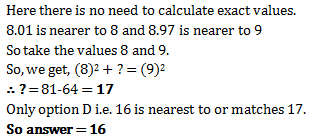QUESTION: 2

### The square of 23 is :

Solution:

Yes the correct option is A because..... 23×23=529.....

QUESTION: 3

### The square of which of the following would be even number?

Solution:

Since the square of an odd natural number is odd and that of an even number is an even number.

∴    (i)  The square of 431 is an odd number

(∵  431 is an odd number)

(ii)   The square of 2826 is an even nnumber.

(∵ 2826 is an even number)

(iii) The square of 7779 is an odd number

(∵ 7779 is an odd number)

(iv)  The square of 131 is an odd nnumber.

(∵ 82004 is an odd number)

QUESTION: 4

The square of which of the following would be odd number?

Solution:

Squares of odd numbers are always odd

QUESTION: 5

Which of the following is a perfect square ?

Solution:

81 is the square of 9..... 9 × 9 = 81

QUESTION: 6

What will be the one digit in the square of 1234

Solution:

One digit will be 6 because 1234
4, is at he last and 4² = 16.
So, 6 is at the last.

QUESTION: 7

What will be the number of zeros in the square of 400 ?

Solution:

Square of 400 = 160000

Therefore, the number of zeroes is 4

QUESTION: 8

The perfect square number between 30 and 40 is  :

Solution:

Since 35,32,39 are not perfect squares . hence the only perfect square between 30 and 40 is 36

QUESTION: 9

By what least number should we multiply 1008 to make it a perfect square?

Solution: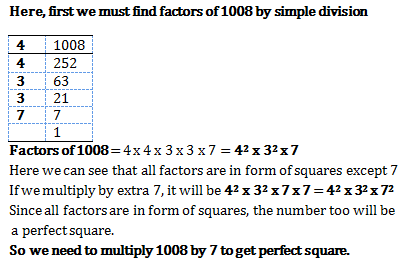QUESTION: 10

Sum of squares of two numbers is 145. If square root of one number is 3, find the other number.

Solution: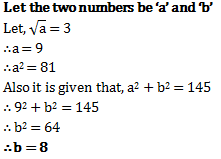QUESTION: 11

Which of the following number would have digit 1 at units place ?

Solution:

The squares that have 1 and 9 at their unit place, ends with 1.

IN this case, 81will end in 1

QUESTION: 12

How many natural numbers lie between 92 and 102?

Solution:

Between 92 and 102

Here, n = 9 and n + 1 =10

:. Natural number between 92 and 102  are (2 x n) or 2 x 9, i.e. 18. hence,correct option is (c).

QUESTION: 13

How many non square numbers lie between 11and 122?

Solution:

Between 112 and 122

Here, n = 11 and n + 1 = 12

∴ Natural numbers between 112 and 122 are (2 × n) or 2 × 11, i.e. 22.

QUESTION: 14

Which of the following are the factors of ac+ ab + bc + a2

Solution:

The question should be a2+ac+bc+ab
=a(a+c)+b(a+c)=(a+c)(a+b)

QUESTION: 15

How many numbers lie between square of 12 and 13?

Solution:

The number of non square numbers
between n² and ( n + 1 )² is 2n
Here,
n = 12,
n + 1 = 13
Therefore ,
Number of natural numbers lie
between 12² and 13² = 2 × 12
= 24

QUESTION: 16

Which of the following arc the factors of 1 – x2?

Solution:
QUESTION: 17

What is the number of digits in the square root of 390625?

Solution:

Square root of 390625=625.Hence its a 3 digit number

QUESTION: 18

The square root of 6400 is

Solution: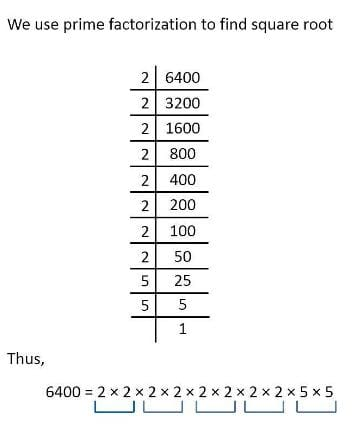Therefore, the square root is 80

QUESTION: 19

By which smallest number 90 must be multiplied so as to make it a perfect square ?

Solution:

By prime factorisation we have 90=2*3*3*5, so the least number by which 90 should be multiplied is 2*5=10

QUESTION: 20

By which smallest number 48 must be divided so as to make it a perfect square ?

Solution:

By Prime factorisation, we have 48=2*2*2*2*3. We have two pairs of 2 but no pair of 3. Hence 48 must be divided by 3 to make it a perfect square.

QUESTION: 21

Which smallest number should be added to 80 so as to make it a perfect square ?

Solution:

The smallest number to be added to 80 so as to obtain a perfect square number is 1.

80+1=81 and the square root of 81 is 9,so 81 becomes a perfect square.

QUESTION: 22

What could be the possible “one’s digit” of the square root of 625?

Solution:

625 is square root of 25 and one's digit of 25 is 5

QUESTION: 23

Which is the smallest three-digit perfect square?

Solution:

Yes as the the smallest 3 digit no. is 100 and it is the square of 10 so also a perfect square.

QUESTION: 24

Which is the greatest three-digit perfect square?

Solution:

We know that the largest 3 digit number is 999. So we will find out the square root of 999 by long division method and check whether it is a perfect square or not.
We will see that 999 is not a perfect square as it leaves 38 as remainder.

So if 38 will be added to 999 then it will become a 4 digit number. Therefore to find the largest 3 digit perfect square we will subtract 38 from 999.

999 - 38 = 961.

Hence 961 isthe largest 3 digit perfect square whose square root is 31.

QUESTION: 25

Which is the greatest 4-digit perfect square?

Solution:

By division method we find the root of 9999 and we get the remainder 198.So subtracting 198 from 9999 we get 9801 as the greatest 4-digit perfect square.

QUESTION: 26

Which is the smallest 4-digit perfect square?

Solution:

Apply division method on 1000, gives 3*3=9 leaves remainder 1 .Then get the two zeros, which makes it 100 then you find the next number ie,62*2=124 and then subtract 100 from 124 you get 24 which is to be added in 1000. Hence it’s 1024

QUESTION: 27

What will be the number of digits in the square root of 25600?

Solution:

The square root of 25600 is 160. Hence, number of digits is 3.

QUESTION: 28

What will be the number of digits in the square root of 1296?

Solution:

The square root of 1296 is 36.

Hence, number of digits os 2

QUESTION: 29

The square root of 12.25 is _______________ .

Solution:

The square root of 12.25 is 3.5

QUESTION: 30

What is the length of the side of a square whose area is 441 cm2 ?

Solution:

We know area of a square =a^2 given area =441cm^2 so a^2=441cm2 a^2=(21cm)^2 so a=21...length of square =21cm

QUESTION: 31

In a right angle triangle ABC, right angled at B, AB = 6cm, BC = 8cm ,then AC = ____ .

Solution:

We have (AB)2+(BC)=( AC)2 which is (AC)= 36+64 . Hence AC = 10cm.

QUESTION: 32

Which least number should be subtracted from 629 so as to get a perfect square ?

Solution:

If we subtract 4 from the given number, then it becomes 625.

625 is a square of 25.

QUESTION: 33

The square root of 1.21 is

Solution: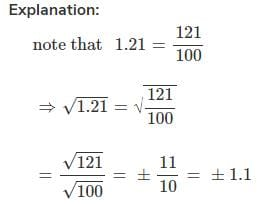QUESTION: 34

What is the smallest square number which is divisible by each of the numbers 6,9 and 15 ?

Solution:

LCM of 6,9 and 15 is 90. So any multiple of 90 will be divisible by 6,9 and 15. The only square number in the options is 900, so 900 is the smallest square number which is divisible by 6,9 and 15.

QUESTION: 35

The square of 1.2 is

Solution:

Required square of 1.2 is 1.44

QUESTION: 36

The square root of 169 is

Solution:

13 is the square root of 169.

QUESTION: 37

What is the length of the diagonal of a rectangle having dimensions 3 cm and 4 cm?

Solution: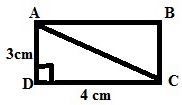Here let us consider ABCD is a rectangle given in the figure.

DC = 4 cm (Length)

To Find:

AC (Diagonal)

We know that, Triangle ADC is a right triangle, right angled at D. So by using Pythagorus Theorem

Length^2 + Breadth^2 = Hypotenuse^2     (diagonal)

=> 4^2 + 3^2 = AC^2

=> 16 + 9 = AC^2

=> 25 = AC^2

=> AC = √25 = 5

Therefore the length of the diagonal AC is 5 cm.

QUESTION: 38

What will be the length of third side of a right angled triangle whose hypotenuse is 5cm and one of the side is 3 cm ?

Solution:

By using Pythagoras theorem
H= P+ B2
Third side =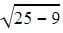= 4cm

QUESTION: 39

Which of the following is not a perfect square ?

Solution:

Squares of all integers are known as perfect squares. All perfect squares end in 1, 4, 5, 6, 9 or 00 (i.e. Even number of zeros). Therefore, a number that ends in 2, 3, 7 or 8 is not a perfect square.

QUESTION: 40

What is the difference between 1500 and nearest perfect square number smaller than 1500?

Solution:

We know that 402 = 1600

So we must start finding squares of numbers less than 40

Take 39 first

402 = 1600, :. 1600 -1500 = 100

392 = 1521,  :.1521 -1500 = 21

382= 1444, .: 1500 -1444 = 56

Thus nearest perfect square is 392.

But there is no option of difference of 21.

so we'll go the next available nearest possible that is 1444 i.e 382

So answer is 1500-1444 = 56Use Code STAYHOME200 and get INR 200 additional OFF Use Coupon Code